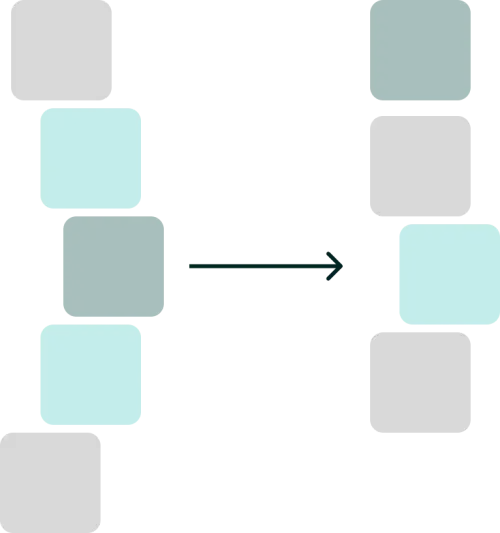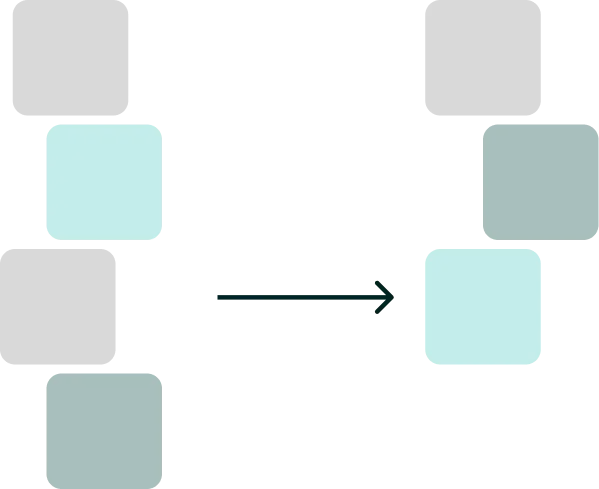# Deep nesting - Why and how to avoid

21/01/2023

... if you need more than 3 levels of indentation, you're screwed anyway, and should fix your program.

This is written in the Linux style guide. Let's see why they have that rule and how we can overcome deeply nested code. Oh yeah and by the way the Linux style guide also states that they use 8 spaces for indentation. If I would do that with my example code you might have to scroll.

Have a look at the following code:

``````
// Here we are at the first level of indention
double CalculateRate(List<Order> orders, Customer customer)
{
// Here we are at the second level of indention
if (orders.Count > 0)
{
// Here is the third level
double discountRate = 1d;
{
// And here the forth level
discountRate = 0.95d;
}
else if (orders.Length > 1000)
{
discountRate = 0.90d;
}

return orders.Sum(s => s.Price * discountRate)
}
else
{
return 0d;
}
}
``````

This method has 4 levels of indention and it is hard to keep track of what is going on here. What branch is the most important one??What are edge cases? Where should I focus? Well, let's refactor that thing. I will show you two methods to do so: Extraction and Inversion.

## Extraction

We can make the function simpler by extracting parts of the logic into a separate function. Ideally, the function lives in the domain object that handles the logic.``````double GetDiscountRate(List<Order> orders, Customer customer)
{
{
return 0.95d;
}
else if (orders.Length > 1000)
{
return 0.9d;
}
else
{
return 1d;
}
}

// Here we are at the first level of indention
double CalculateRate(List<Order> orders, Customer customer)
{
// Here we are at the second level of indention
if (orders.Count > 0)
{
// Here is the third level
double discountRate = GetDiscountRate()

return orders.Sum(s => s.Price * discountRate);
}
else
{
return 0d;
}
}
``````

Okay, a bit better. We are down to 3 levels as we wanted, but we can go further with the next technique.

## Inversion

If we check the edge cases first and return early. We can achieve this by inverting our if statements. We don’t need an else after a return statement. Also, the usage of ternary operators can reduce nesting.? Be careful, sometimes early returns or ternary operators?can make things less readable.``````// Here we are at the first level of indention
double CalculateRate(List<Order> orders, Customer customer)
{
if (order.Count == 0)
{
return 0d;
}

// Here we are at the second level of indention
double discountRate = GetDiscountRate(orders, customer);
return orders.Sum(s => s.Price * discountRate);
}

double GetDiscountRate(List<Order> orders, Customer customer)
{
{
return 0.95d;
}

return order.Length > 1000
? 0.9d;
: 1d;
}
``````

## Conclusion

With Extraction and Inversion (plus ternary operators), we can reduce nesting a lot. The main advantage is that it is obvious to the reader what is important! We have a clear flow. If we are interested in details, we can jump into the method. So overall, we reduced the mental complexity of our method!

7
An error has occurred. This application may no longer respond until reloaded. Reload x